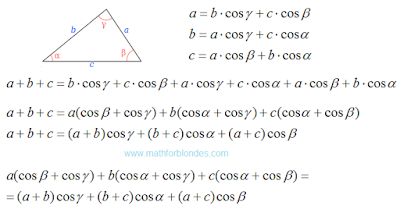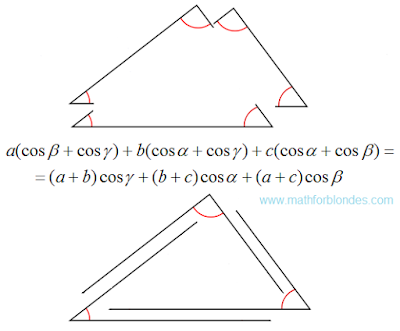## 3/14/2016

### Law of cosines for perimeter

Carefully look at the proof of the law of cosines and make some corrections.Analysis of proof

Now we can write the law of cosines for the perimeter of the triangle.Law of cosines for perimeter

The result is a very simple and beautiful formula that describes the entire triangle. The law of cosines shows a relationship between the angles and the lengths of the sides of a triangle.The geometry of the law of cosines

Law of cosines for perimeter describes the perimeter of the triangle made up of one-dimensional Euclidean spaces. For multidimensional spaces cosine law has a different view.